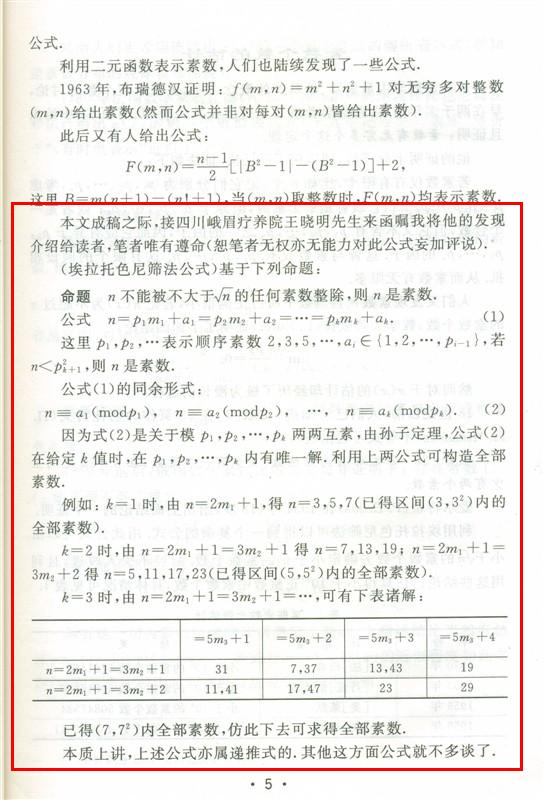• 汇集公众科学智慧交流科学思想见解
• 点燃科学智慧火花构建互动交流平台## 利用抽屉原理证明素数无穷多

1，如果n是合数，则它有一个因子d满足1<d≤2，若自然数n是一个素数，当且仅当它不能被不大于任何素数整除，则n是一个素数。）。.........(1)..........（2）

k=2时，，解得n=7，13，19；，解得n=5，11，17，23。求得了（5，）区间的全部素数。

 k=3时31 7,37 13,43 1911,41 17,47 23 29）（）()...（）....（3）个解.|---------------已经筛过部分----------------------|------------未经筛过部分------------|。

【1】将1至...按照为一组，划分成...个组（或区间）

[1，]； [$p_{{k-1}}p_{{k}}+1$，2]； ....； [...—（）+1，...]。

【2】把（1）式（2）式的解数即（3）式（）（）()...（）当做抽屉。

【3】由于假定了（1）式（2）式没有小于$p^{2}_{k}$的解，第一区间就没有解，因为<$p^{2}_{k}$...$p_{k-2}-1$) k < （...）k < （）（）()...（）...（4）

【4】 对比（4）式$\frac{P_{1}-1}{1}\cdot$$\frac{P_{2}-1}{P_{1}}\cdot\frac{P_{3}-1}{P_{2}}\cdot\frac{P_{4}-1}{P_{3}}\cdot\frac{P_{5}-1}{P_{4}}\cdot\frac{P_{6}-1}{P_{5}} \cdots \frac{P_{K-1}-1}{P_{K-2}} \cdot\frac{P_{K}-1}{K} \cdot$

【5】由于抽屉的数量（）（）()...（）是由孙子定理给出的，而装进抽屉的物品（比如乒乓球）.......$p_{k-2}-1$,) k少于抽屉，至少有一些抽屉是空的，这样就违反了孙子定理，与孙子定理矛盾，必然是错误的。所以，原先假设最后一个素数是，显然是错误的，素数无穷多个。